#Function Repository Resource:

# RandomRorschach

Generate random Rorschach tests

Contributed by: Anton Antonov
 ResourceFunction["RandomRorschach"][] makes a random Rorschach test image. ResourceFunction["RandomRorschach"]["Graphics"] makes a random Rorschach test graphics object. ResourceFunction["RandomRorschach"]["HalfGraphics"] makes the left half of a random Rorschach test graphics object. ResourceFunction["RandomRorschach"]["Image"] makes a random Rorschach test image. ResourceFunction["RandomRorschach"]["HalfImage"] gives the left half of a random Rorschach test image.

## Details and Options

ResourceFunction["RandomRorschach"] takes a string argument that is expected to be one of "Graphics", "HalfGraphics", "Image" or "HalfImage".
By default, an image is returned (i.e. "Image" is the default value of the first argument).
With "Image", ResourceFunction["RandomRorschach"] makes a random filled scribble seed, reflects the seed over the right bounding box boundary and transforms the result with image effects.
With "Graphics", ResourceFunction["RandomRorschach"] does not apply image effects.
ResourceFunction["RandomRorschach"] takes all options of Rasterize and the resource function RandomScribble.
In addition, ResourceFunction["RandomRorschach"] takes the options "ImageEffects" and "CropSeed".
The option "ImageEffects" takes as a value a list of "second arguments" of ImageEffect or image manipulation functions that return images as results.
ResourceFunction["RandomRorschach"] has its own default values for the RandomScribble options "NumberOfStrokes", "OrderedStrokePoints" and "ConnectingFunction".
Here are the ResourceFunction["RandomRorschach"]-specific options and their default values:
 "NumberOfStrokes" Default number of strokes in the scribble seed "OrderedStrokePoints" False whether to order the scribble stroke points or not "ConnectingFunction" connecting function for the scribble strokes "ImageEffects" Default image effects to be applied "CropSeed" True whether to crop the seed or not

## Examples

### Basic Examples (2)

Make a random Rorschach image:

 In:=Out=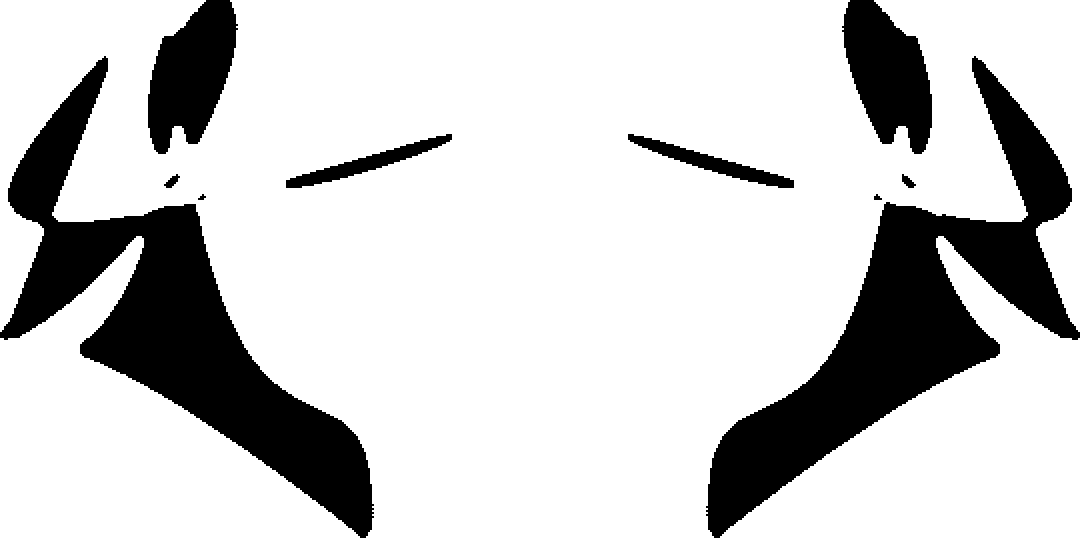Make a random Rorschach graphics object:

 In:=Out=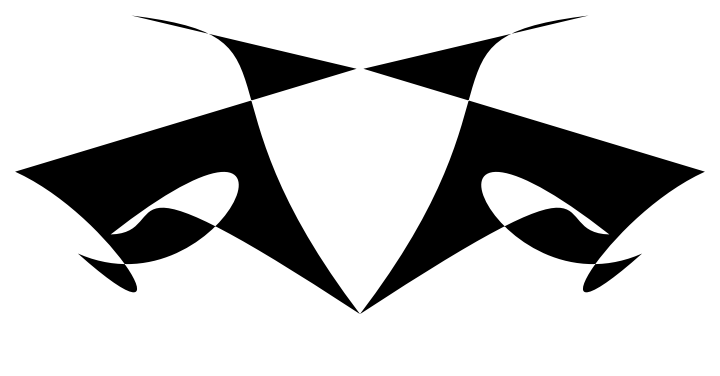### Scope (2)

RandomRorschach takes a string argument that is expected to be one of "Graphics", "HalfGraphics", "Image" or "HalfImage". Here is a table with corresponding results:

 In:=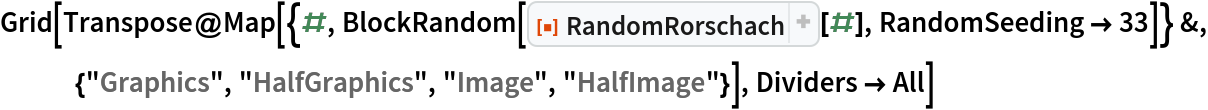Out=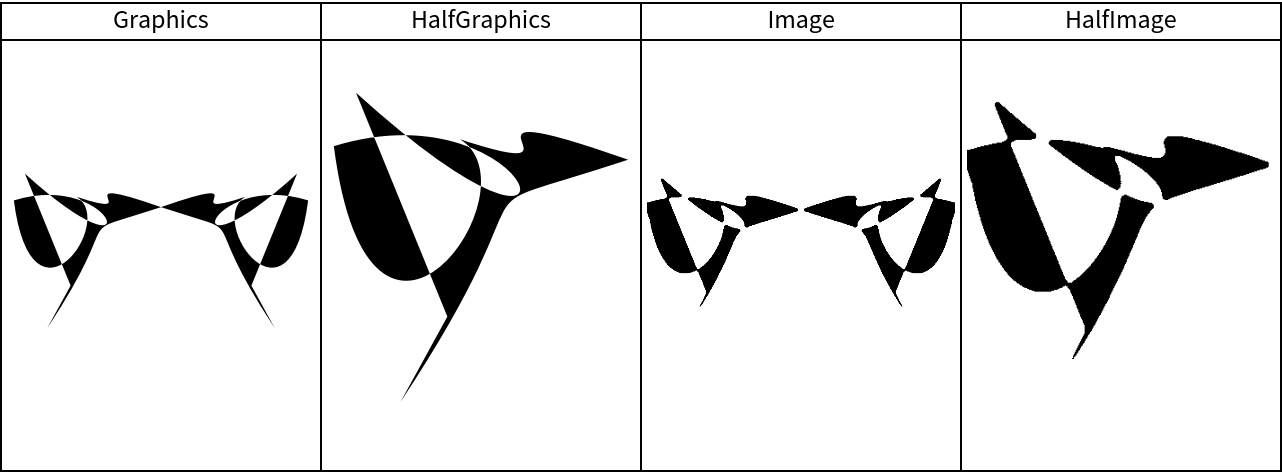The RandomRorschach results are controlled using options:

 In:=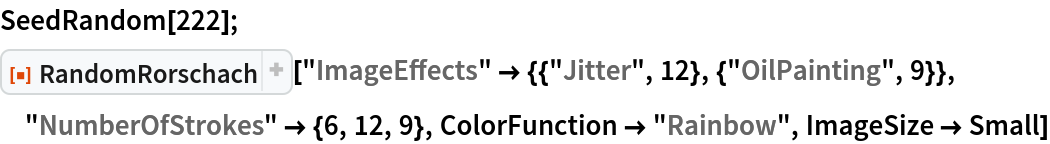Out=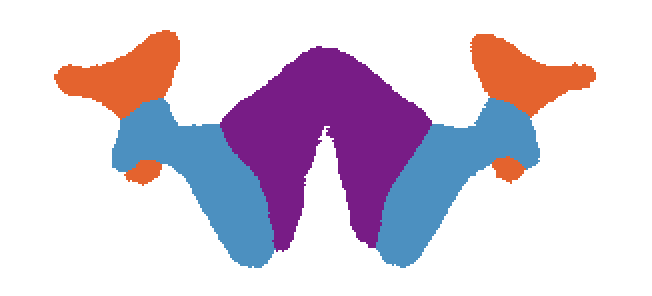### Options (5)

#### CropSeed (1)

The option "CropSeed" specifies whether the seed is cropped (from its right side):

 In:=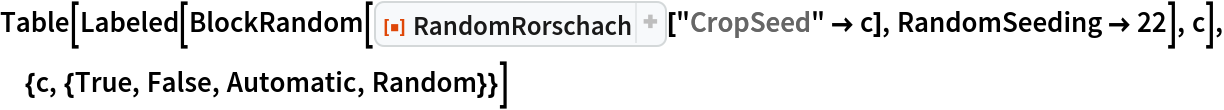Out=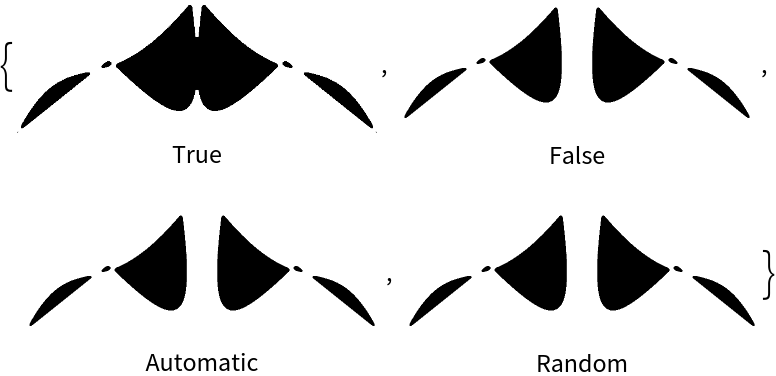If the values Automatic or Random are given, then a logical value is randomly selected.

#### ConnectingFunction (1)

The value of the option "ConnectingFunction" is passed to RandomScribble. Here are examples:

 In:=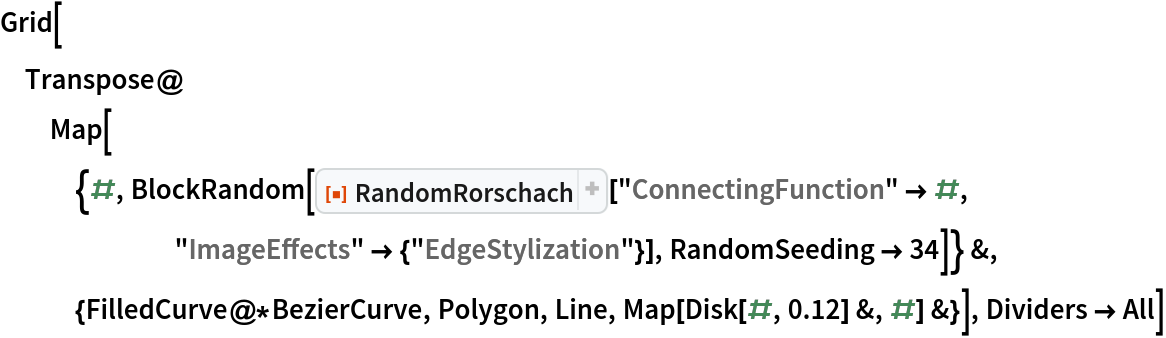Out=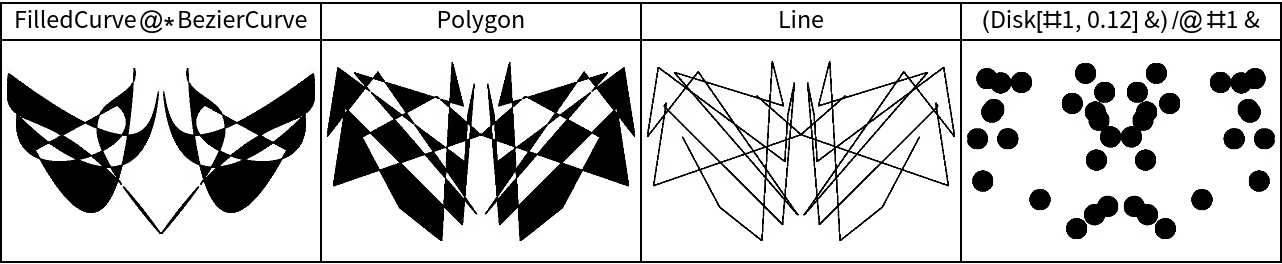#### ImageEffects (1)

The option "ImageEffects" can take a sequence of ImageEffect specs and other image manipulation functions:

 In:=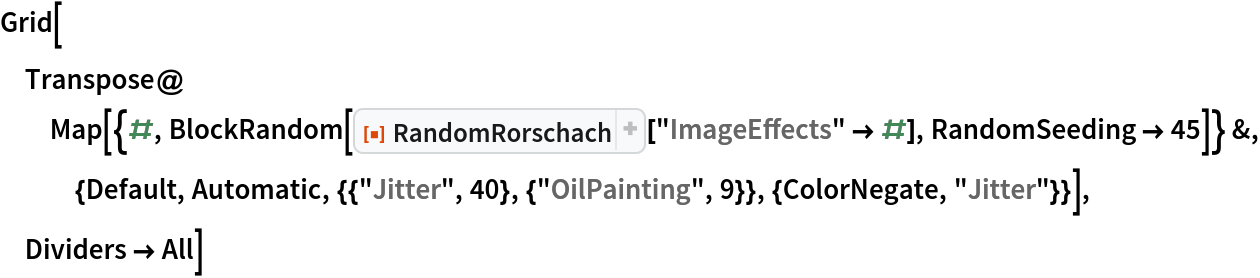Out=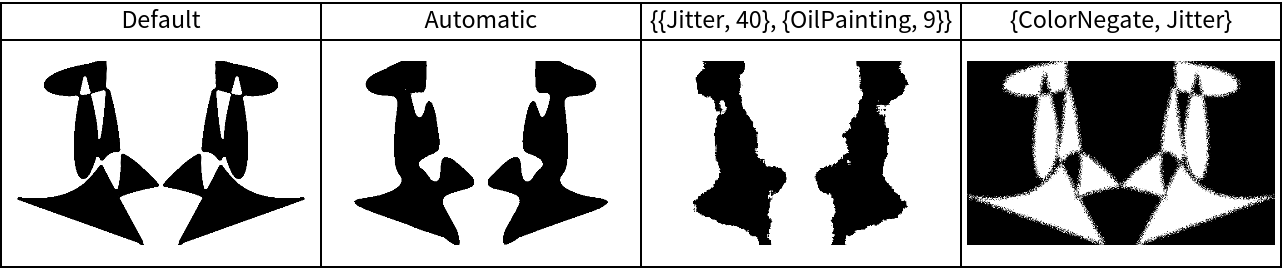If the value is Default, then the value is {{"OilPainting",5}}.

If the value is Automatic, then the code RandomChoice[{{"Jitter",10},"EdgeStylization",{"OilPainting",16}},2] is used.

#### NumberOfStrokes (1)

The value of the option "NumberOfStrokes" can be Default, Automatic, a positive integer or a list of positive integers. It is passed to RandomScribble. Here are examples:

 In:=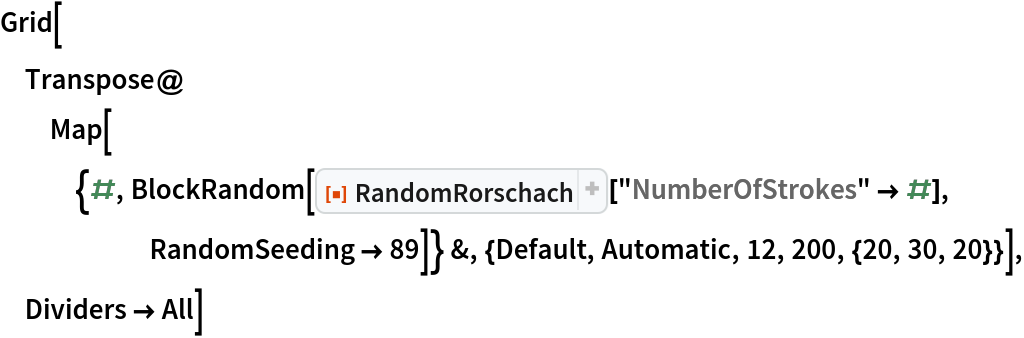Out=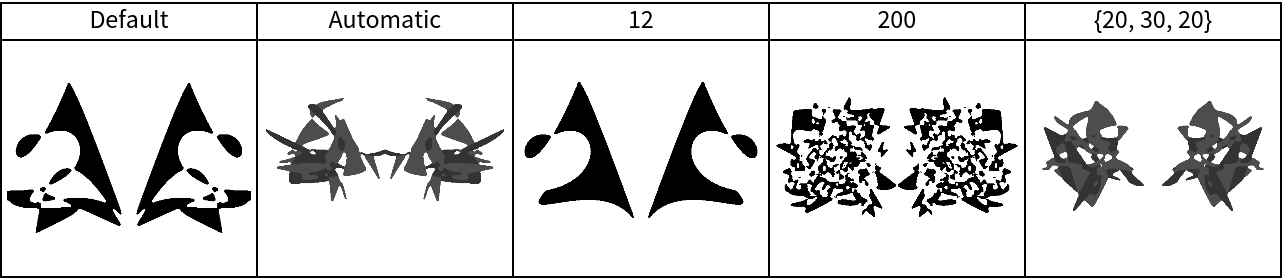If the value is Default, then the code RandomInteger[{6,20}] is used.

If the value is Automatic, then the code RandomInteger[{5,20},3] is used.

#### OrderedStrokePoints (1)

The option "OrderedStrokePoints" takes a logical value. The value is passed to RandomScribble. Here are examples:

 In:=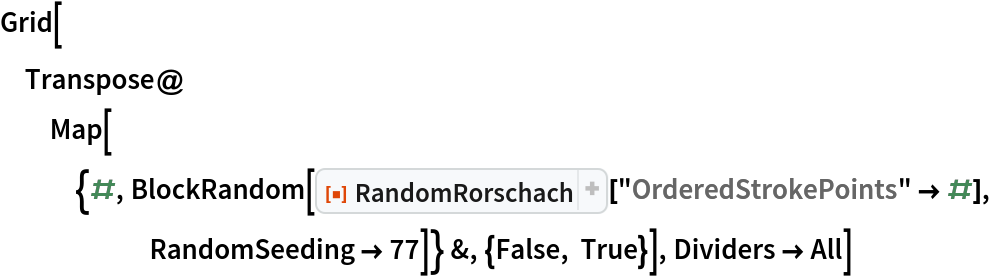Out=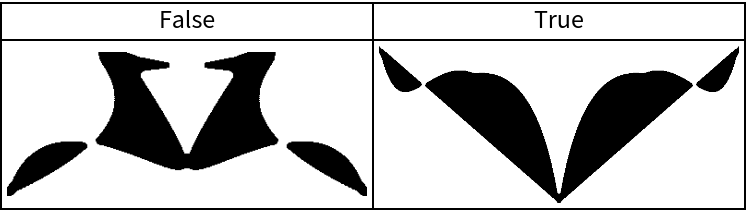### Properties and Relations (3)

Using different color functions would, generally, produce different Rorschach interpretations. Here is a set of Rorschach test images:

 In:=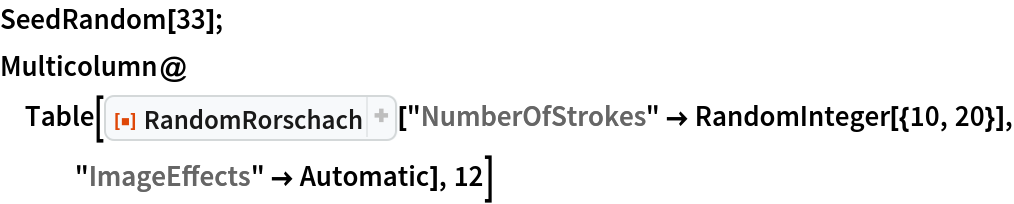Out=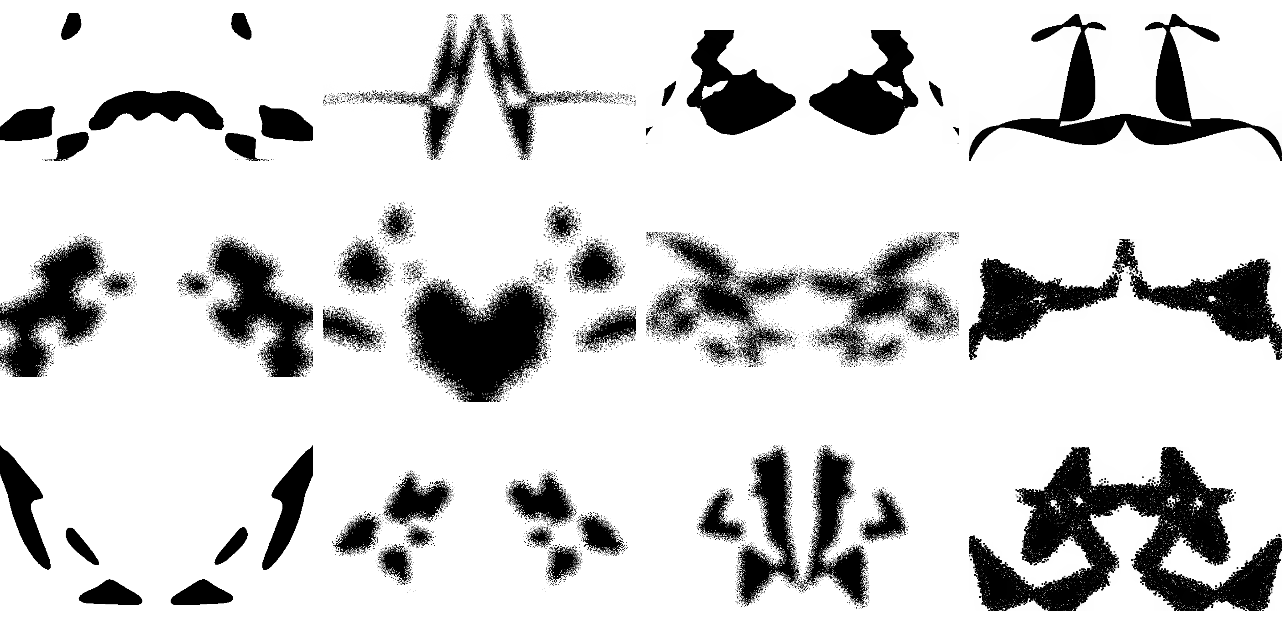Here is another set of images with the same random seed and image effects as shown previously and a different color function:

 In:=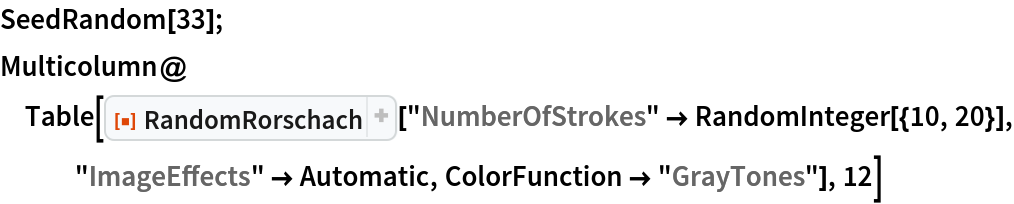Out=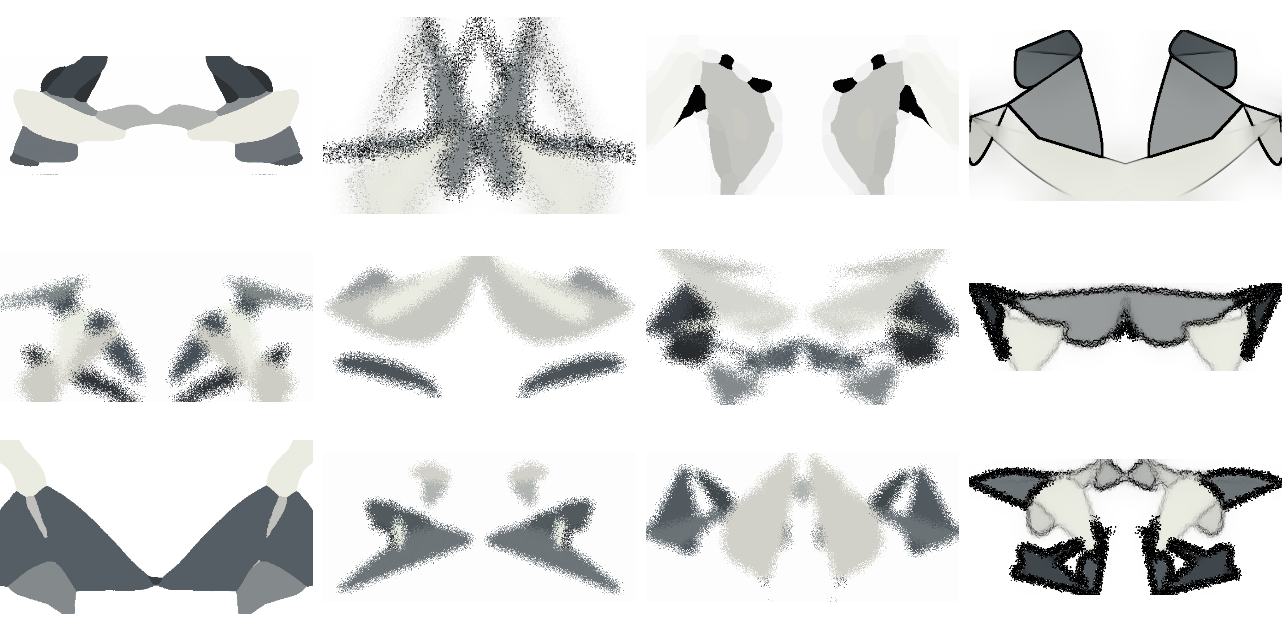In some sense, the option "ImageEffects" is redundant because similar behavior can be achieved with standard Wolfram Language code. Nevertheless, using that option provides a quicker (and shorter) way to get desired results. Here is a Rorschach image creation specification:

 In:=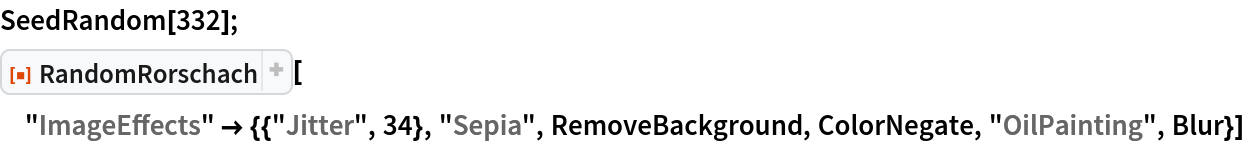Out=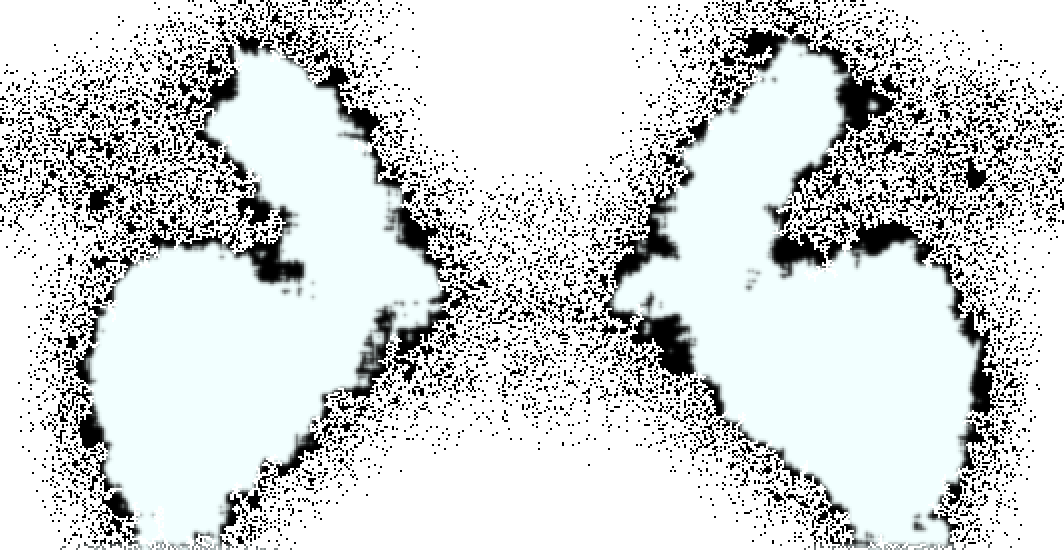Here we generate the Rorschach "initial" image first and then modify it with the functions that correspond to the "ImageEffects" specification above:

 In:=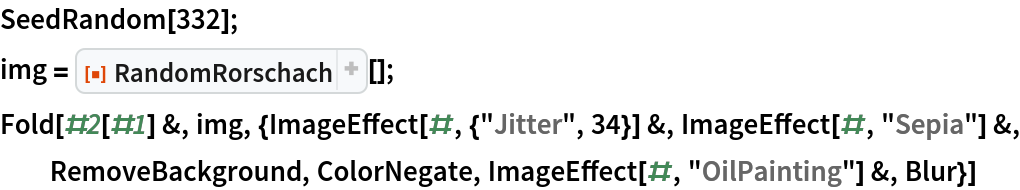Out=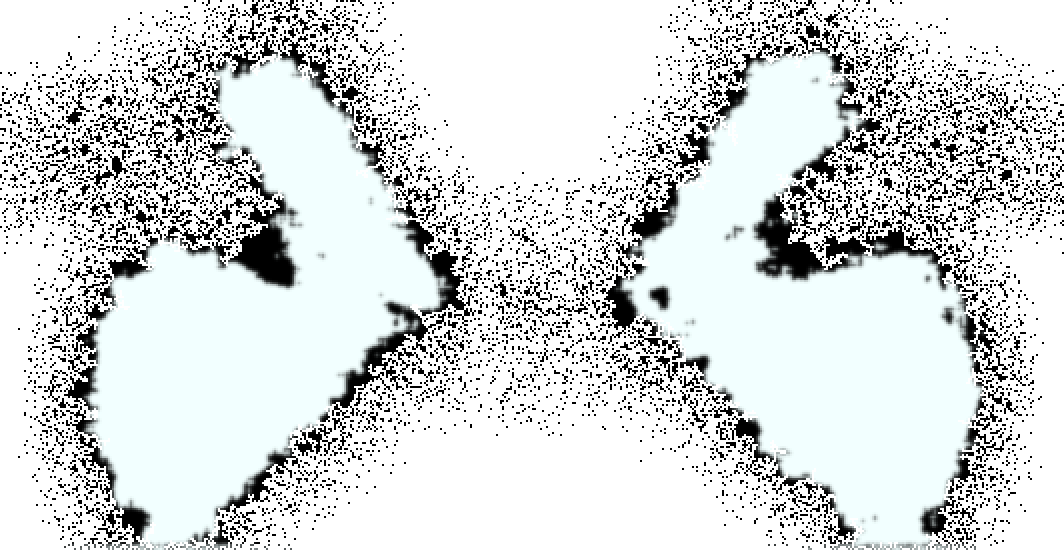The repository functions RandomScribble and RandomMandala are closely related to RandomRorschach:

 In:=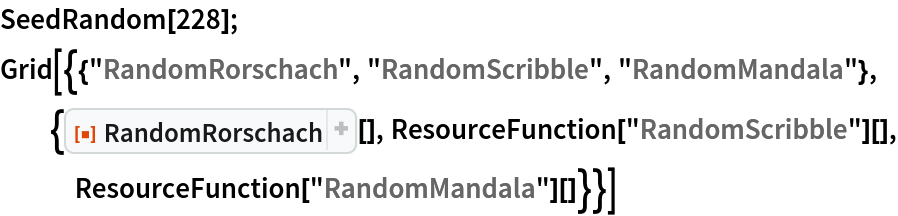Out=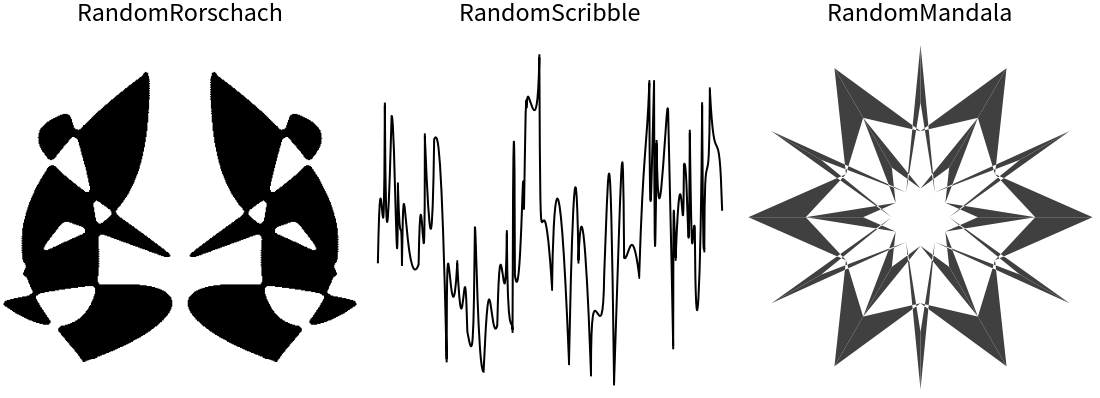### Possible Issues (2)

The values of the option "ImageEffects" might contain wrong second arguments for ImageEffect. In those cases, errors of ImageEffect are shown and the result is \$Failed:

 In:=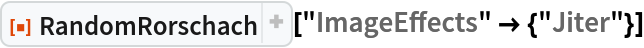Out=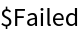\$Failed is also returned if an element of the list is not a function that gives an image result:

 In:=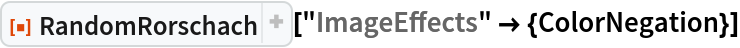Out=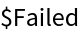### Neat Examples (4)

A table of random Rorschach test images:

 In:=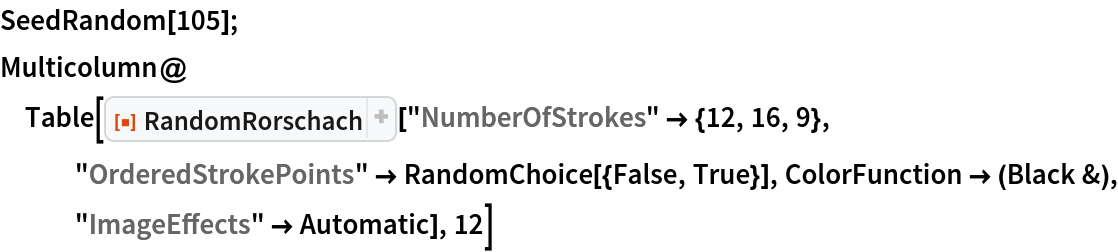Out=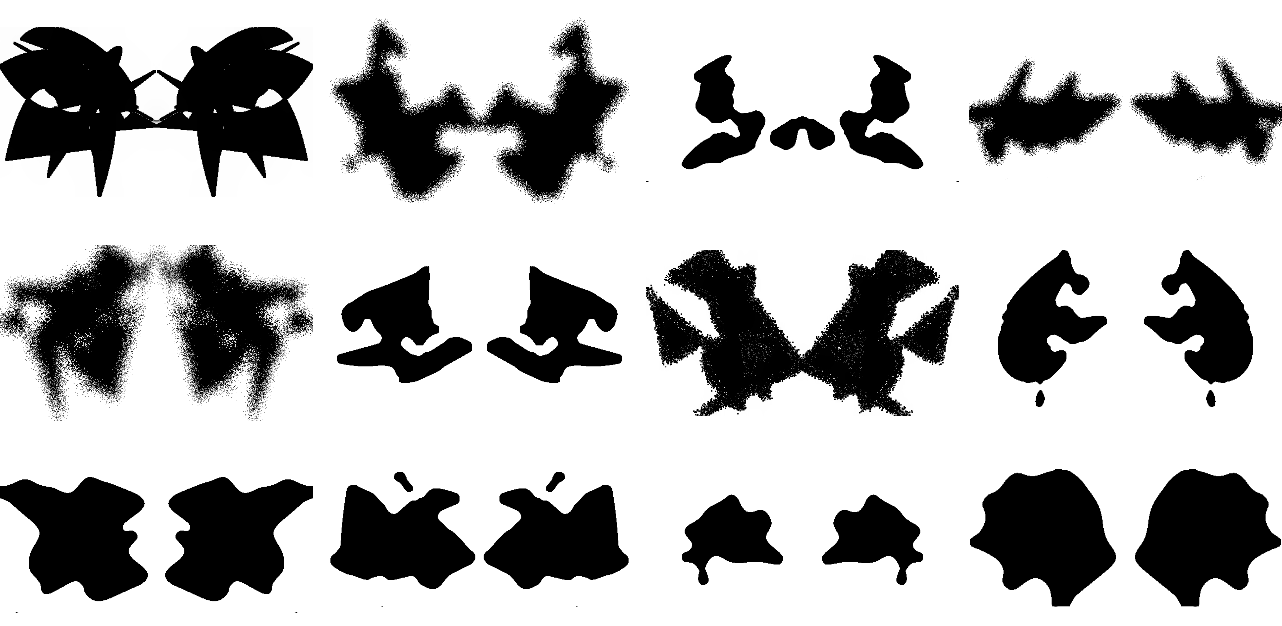Another table with the same random seed as shown previously but with a different color function and image effects:

 In:=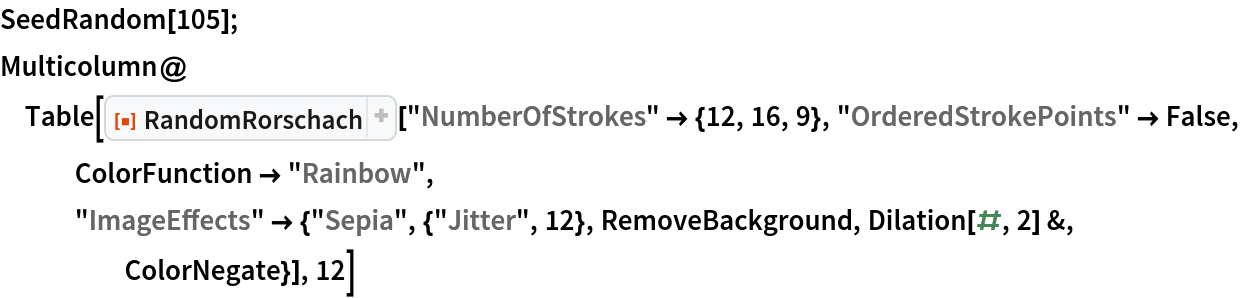Out=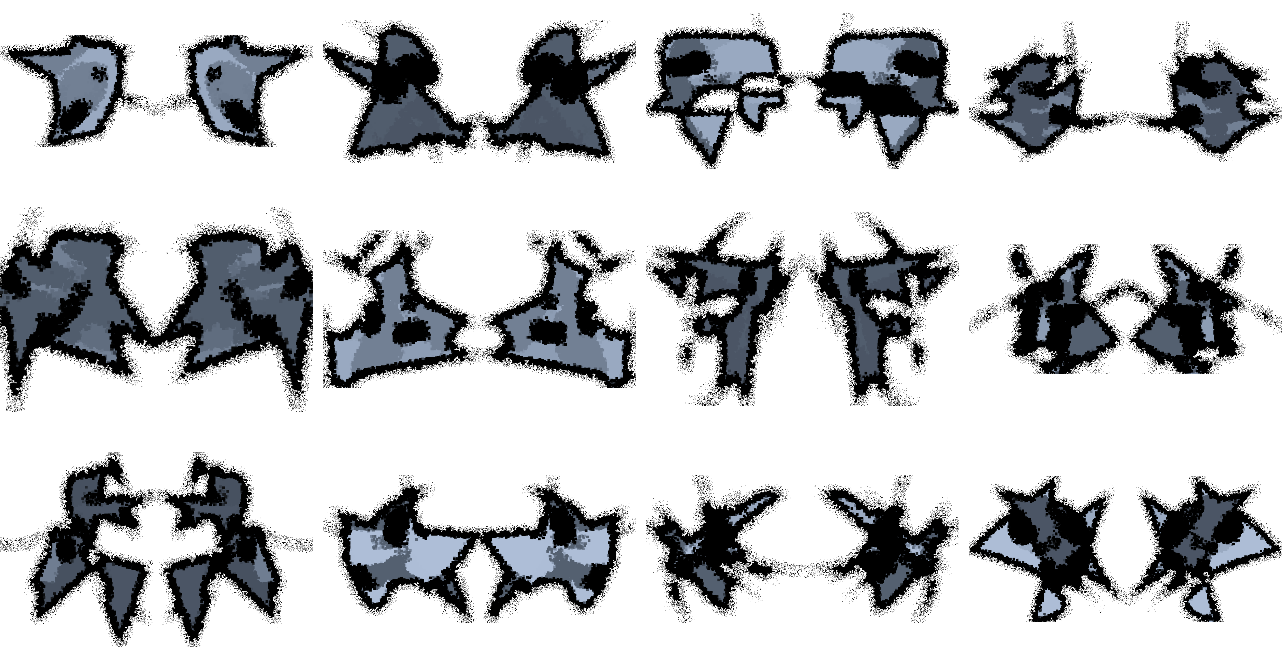"Poodles", or "frogs", or an "electric bolts guy":

 In:=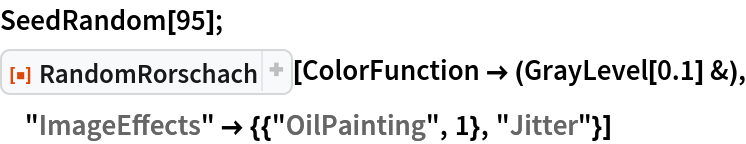Out=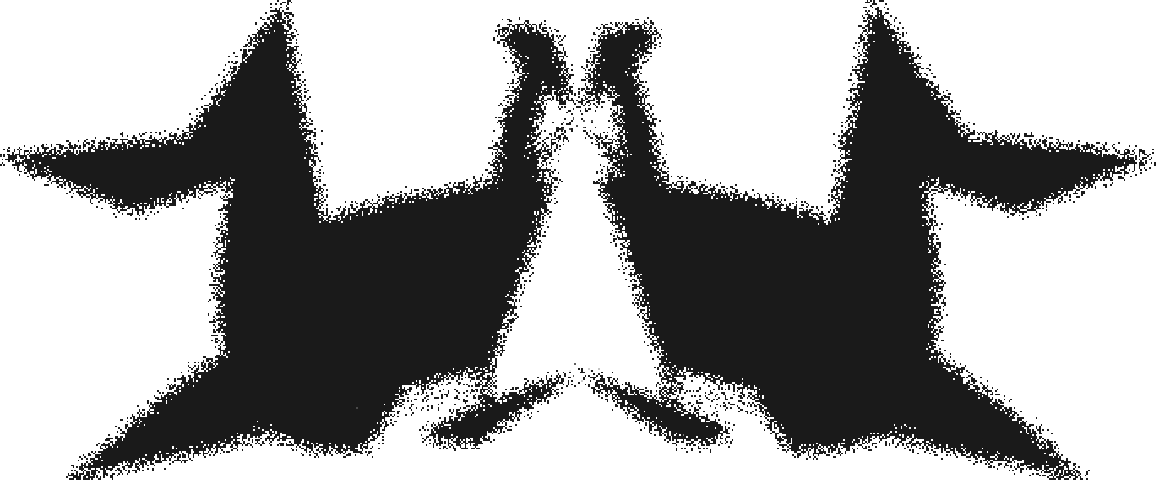The resource function XKCDConvert can be applied to the graphics results of RandomRorschach:

 In:=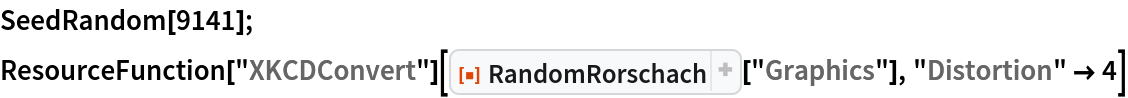Out=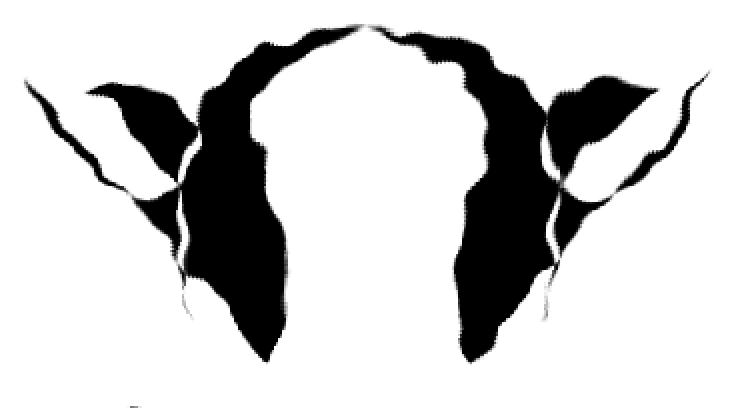Anton Antonov

## Version History

• 1.0.0 – 22 April 2022